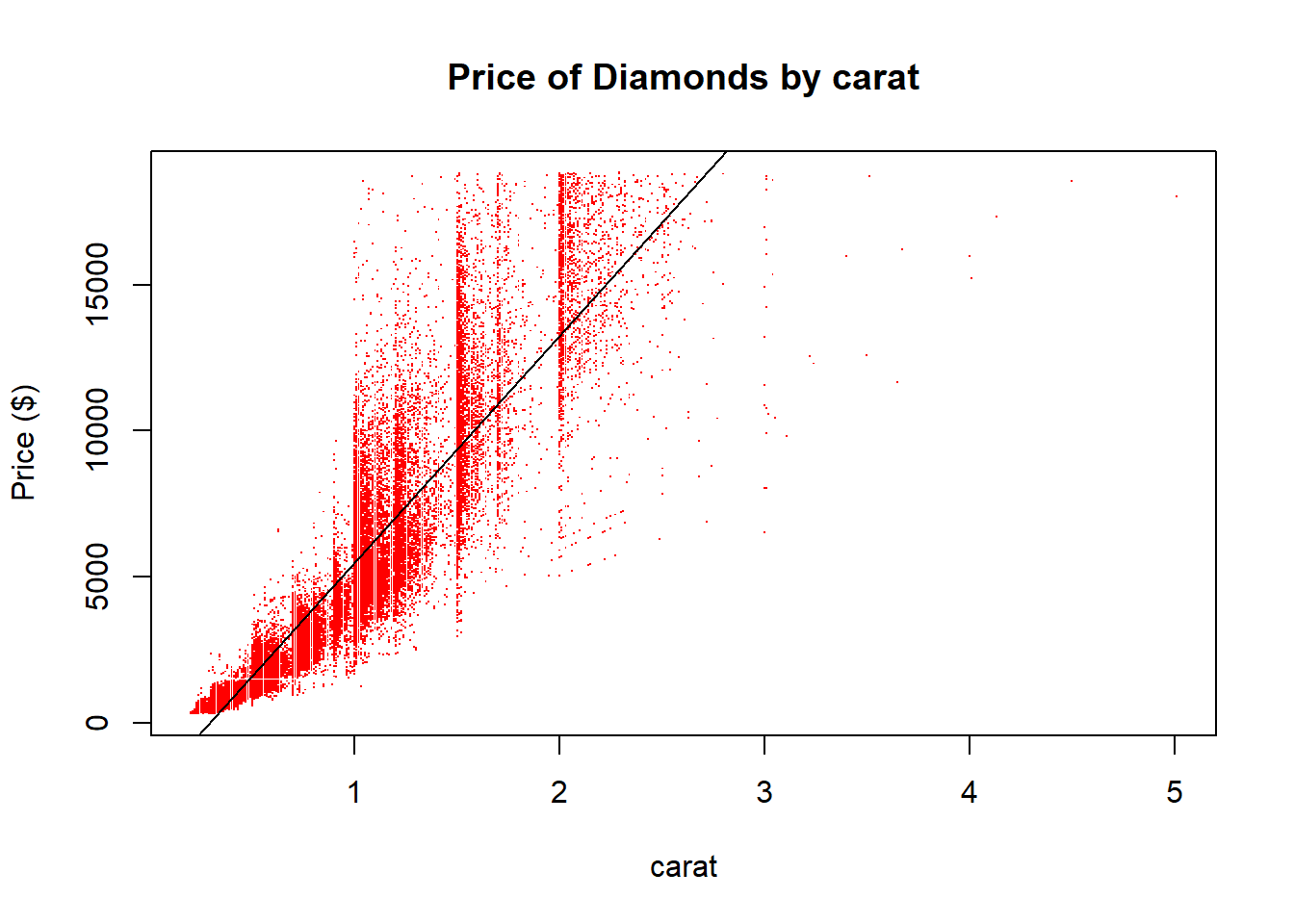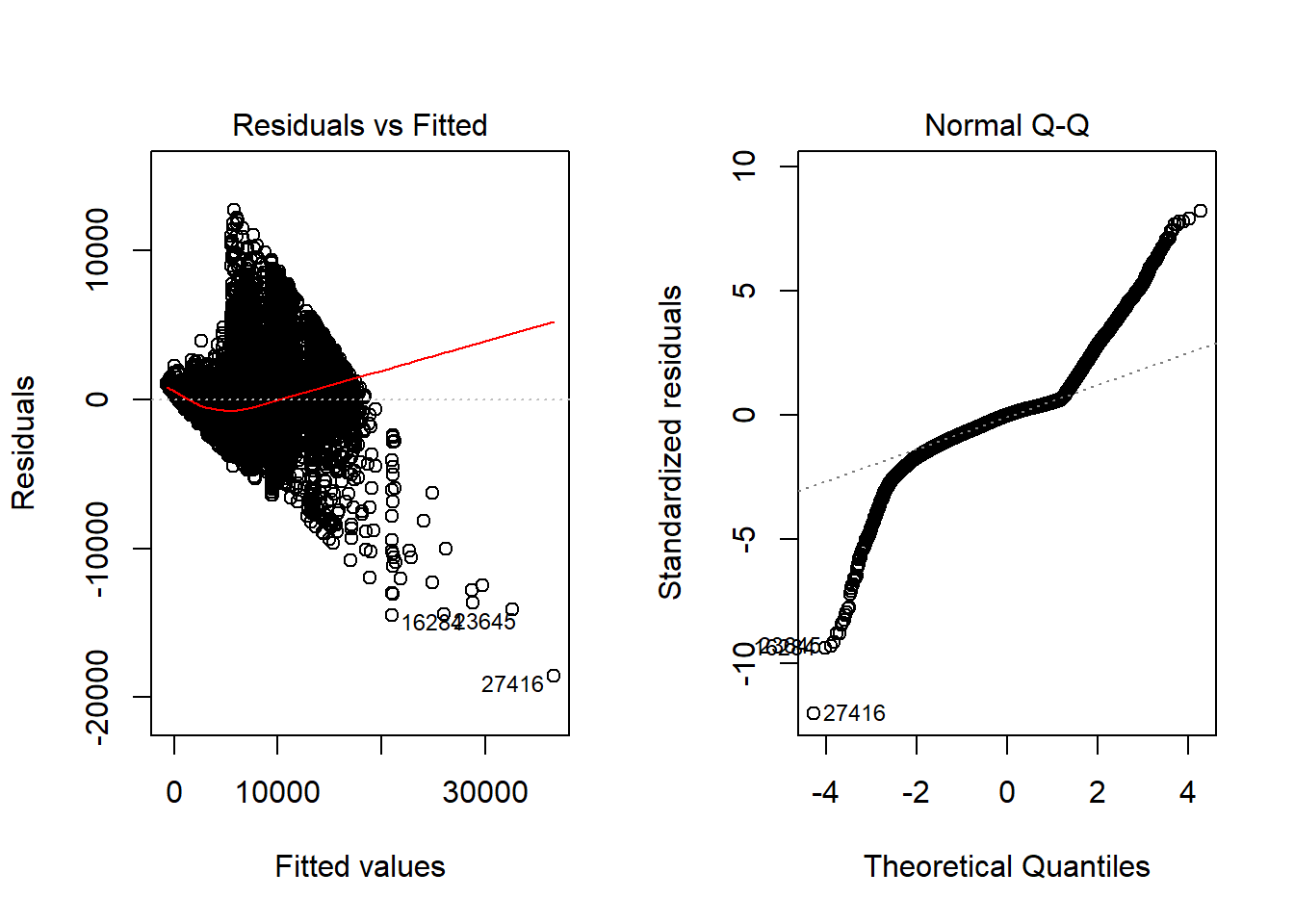### BACKGROUND

Every diamond is a miracle of time and place and chance. Like snowflakes, no two are exactly alike. GIA created the first, and now globally accepted standard for describing diamonds: Color, Clarity, Cut, and Carat. The four aspects factor into the overall quality and worth of a diamond. We want to focus on the the carat aspect, which we can assume is the most significant. Diamond carat weight is the measurement of how much a diamond weighs. The question we want to analyze is “Is there a strong positive relationship between the carat weight and the price of diamonds?” The data gathered from the ‘diamonds’ dataset will be used to perform the regression analysis for this project. There are 53,940 entries in the dataset. Below, we can see the first few data:

knitr::kable(diamonds[c(1,3,4,7),])
carat cut color clarity depth table price x y z
0.23 Ideal E SI2 61.5 55 326 3.95 3.98 2.43
0.23 Good E VS1 56.9 65 327 4.05 4.07 2.31
0.29 Premium I VS2 62.4 58 334 4.20 4.23 2.63
0.24 Very Good I VVS1 62.3 57 336 3.95 3.98 2.47

### ANALYSIS

The hypothesis for our study involves the slope ($$\beta_1$$). If the slope is equal to zero, there is no relationship between carat weight and price of diamonds. If the slope is not equal to zero, we can assume there is a relationship between the two. Our hypotheses for this analysis are as follows:

$H_0: \beta_1 = 0$ $H_a: \beta_1 \neq 0$

The scatterplot below displays the observed relationship between carat weight and the price of the diamond.

diamonds.lm <- lm(price ~ carat, data=diamonds)
plot(price ~ carat, data=diamonds, main="Price of Diamonds by carat", ylab="Price ($)", col="red", pch ='.') abline(diamonds.lm)According to the plot, there does appear to be a moderate positive relationship between carat and price of the diamond. Estimate Std. Error t value Pr(>|t|) carat 7756 14.07 551.4 0 (Intercept) -2256 13.06 -172.8 0 Fitting linear model: price ~ carat Observations Residual Std. Error $$R^2$$ Adjusted $$R^2$$ 53940 1549 0.8493 0.8493 Assuming the relationship is linear, the equation of the fitted line shown in the plot above is Y = -2256.36 + 7756.43 X A t test of the hypotheses stated above about the slope shows the slope is significantly different from zero ($$p$$ $$<$$ $$2.2e-16$$). Hence, we can conclude that the relationship between carat and price is significant. ### INTERPRETATION The estimated value of the slope is 7,756.43 which suggests that: on average, as the weight of the diamond increases one carat, the price of the diamond increases$7,756.43. A 95% confidence interval for the true slope is (7728.8550116, 7783.9962243) which demonstrates that the value of 1 is not in the interval and would imply that the carat and price of diamonds are not directly proportional.

#### Appropriateness of the Regression

It is arguable whether or not the above analysis is appropriate. In order to determine whether the above regression was appropriate or not, we have to check specific requirements. As shown by the residuals versus fitted values plot, there are some odd patterns visible which is probably safe to assume the requirement for linearity between X and Y is not met. Likewise, the Q-Q Plot suggests that normality of the residuals is questionable as well and cannot assumed to be met. Therefore, we have to question the validity of the findings from our simple linear regression analysis.

par(mfrow=c(1,2))
plot(diamonds.lm, which=1:2)Overall, the significance of the carat weight and price might not be as strong as you would think. Possibly, because of the other C’s of cut, color, and clarity, carat isn’t as strong of an indicator of diamond price. This could have caused the failure to meet all the requirements for the tests and we have to take into consideration the other C’s. Overall, we can safely assume that price of diamonds is not only driven by carat, but other aspects as well.